RadSpreadProcessing is already part of UI for WPF and since Q2 2014, it is also available in the Silverlight, AJAX and WinForms suites. If you are wondering how to deal with your spreadsheets, this post is just for you. This is the first of three blogs that aim to demonstrate how to use RadSpreadProcessing to create a document from scratch. While this post focuses on filling the document with data and using formulas, the following two blogs illustrate how to use styles, number formats, images, filters and export to PDF.

To start with some introduction of RadSpreadProcessing, it is a document processing library that gives you the ability to import and export different spreadsheet formats. Since it has no dependencies on UI dlls, you can use it to create and modify files both on the client and on the server. Currently, with RadSpreadProcessing you can:

• Import and export Excel Microsoft Office Open XML Spreadsheet (.XLSX)
• Import and export Comma Separated Values (.CSV)
• Import and export Tab Separated Values (.TXT)
• Export Portable Document Format (.PDF)

And what can RadSpreadProcessing do? The library supports a number of features including automatic fill of data, protection, history, merged cells, hyperlinks, images, filtering, sorting and many more.

In this series of blog posts we will demonstrate how to create an expense report from scratch. The final result will look like this:In this Part 1 we will mainly focus on creating a workbook, filling in the data and setting up the formulas. First, let’s have a closer look at what data we have and how should we organize it. The document contains expense information for the period January 1, 2014 to March 31, 2014, and each expense record has a department, description, date and amount. Once we display all expenses, we will show the total expenses for each of the departments and also the total expenses for the period.

Before we get started, make sure to add references to the following assemblies in your project:

• Telerik.Windows.Documents.Core.dll
• Telerik.Windows.Maths.dll
• Telerik.Windows.Zip.dll

Let’s start with creating a new workbook instance with a single worksheet. Then, change the name of the worksheet to “Expense Report 2014”:

`Workbook workbook = ``new` `Workbook(); `
`Worksheet worksheet = workbook.Worksheets.Add(); `
`worksheet.Name = ``"Expense Report 2014"``; `

We should also set the header of the main data rows. The following snippet assigns the four headers in row 6 in order to leave some space for the title of the document that will be added later.

`worksheet.Cells[5, 1].SetValue(``"Department"``); `
`worksheet.Cells[5, 2].SetValue(``"Description"``); `
`worksheet.Cells[5, 3].SetValue(``"Date"``); `
`worksheet.Cells[5, 4].SetValue(``"Amount"``);`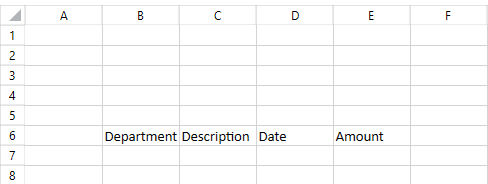Now, it is time to enter the expense data. The following snippet illustrates how to input the information from the ExpenseModel array to the worksheet:

`private` `static` `readonly` `ExpenseModel[] expenses = ``new` `ExpenseModel[] `
`{ `
`    ``new` `ExpenseModel()  `
`    ``{ `
`        ``Description = ``"Accounting and legal"``,  `
`        ``Department = ``"Marketing"``,  `
`        ``Date = ``new` `DateTime(2014, 2, 20), `
`        ``Amount = 610.5953  `
`    ``}, `
`    ``new` `ExpenseModel()  `
`    ``{  `
`        ``Description = ``"Salaries"``, `
`        ``Department = ``"Sales"``, `
`        ``Date = ``new` `DateTime(2014, 1, 3), `
`        ``Amount = 3563.7902  `
`    ``} `
`    ``//... `
`} `

`int` `currentRow = 6; `
` `
`foreach` `(ExpenseModel model ``in` `expenses) `
`{ `
`    ``this``.worksheet.Cells[currentRow, 1].SetValue(model.Department); `
`    ``this``.worksheet.Cells[currentRow, 2].SetValue(model.Description); `
`    ``this``.worksheet.Cells[currentRow, 3].SetValue(model.Date); `
`    ``this``.worksheet.Cells[currentRow, 4].SetValue(model.Amount); `
`     `
`    ``currentRow++; `
`}`

Following these steps the worksheet should look like this: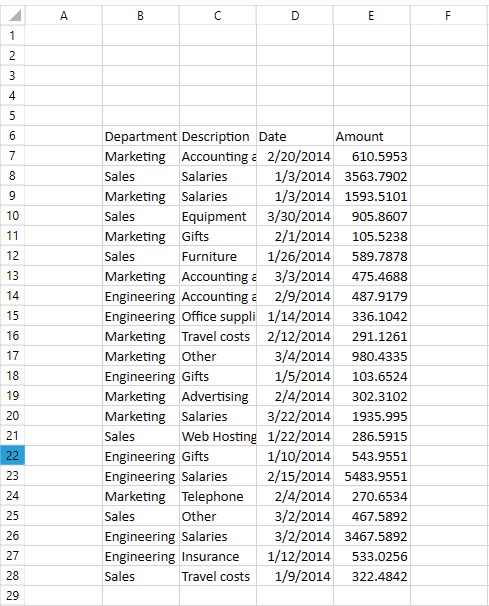Now that all expense data is entered, let’s add a total row for the expenses of each department and one total row for all expenses. To add a total row for each department individually, we can use the SUMIF function and set its criteria to the name of the department. In this way, the function will add only the expenses of the department specified as criteria.

Using the SUMIF formula we can sum only the “Sales” department expenses as shown in the image below: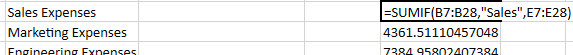To calculate the total expenses of all departments, we can use the SUM function as follows: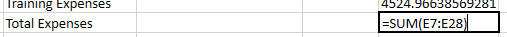The next snippet creates the total rows using the SUM and SUMIF functions:

`worksheet.Cells[28, 1].SetValue(``"Sales Expenses"``);`
`worksheet.Cells[29, 1].SetValue(``"Marketing Expenses"``);`
`worksheet.Cells[30, 1].SetValue(``"Engineering Expenses"``);`
`worksheet.Cells[31, 1].SetValue(``"Total Expenses"``);`

`string` `listSeparator = SpreadsheetCultureInfo.ListSeparator;`
`worksheet.Cells[28, 4].SetValue(``string``.Format(``"=SumIf(B7:B28{0}\"Sales\"{0}E7:E28)"``, listSeparator));`
`worksheet.Cells[29, 4].SetValue(``string``.Format(``"=SumIf(B7:B28{0}\"Marketing\"{0}E7:E28)"``, listSeparator));`
`worksheet.Cells[30, 4].SetValue(``string``.Format(``"=SumIf(B7:B28{0}\"Engineering\"{0}E7:E28)"``, listSeparator));`
`worksheet.Cells[31, 4].SetValue(``"=Sum(E7:E28)"``);`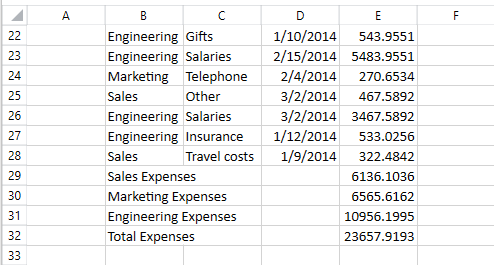Once all expense data is entered, we can add the title of the document in the cells above the expense records. The following code snippet shows how to enter the name of the company, the Expense Report title and the period for which the data is related. Note that in order to autofit the columns containing expense data, we will merge across these values, so that each of them spans over four columns. The code also assigns horizontal alignment.

`CellSelection companyNameCells = worksheet.Cells[1, 1, 1, 4]; `
`companyNameCells.Merge(); `
`companyNameCells.SetValue(``"My Company"``); `
`companyNameCells.SetHorizontalAlignment(RadHorizontalAlignment.Left); `
` `
`CellSelection expenseReportCells = worksheet.Cells[2, 1, 2, 4]; `
`expenseReportCells.Merge(); `
`expenseReportCells.SetValue(``"Expense Report"``); `
`expenseReportCells.SetHorizontalAlignment(RadHorizontalAlignment.Right); `
` `
`CellSelection periodCells = worksheet.Cells[3, 1, 3, 4]; `
`periodCells.Merge(); `
`periodCells.SetValue(``"for the period 1 January 2013 to 31 December 2013"``); `
`periodCells.SetHorizontalAlignment(RadHorizontalAlignment.Right); `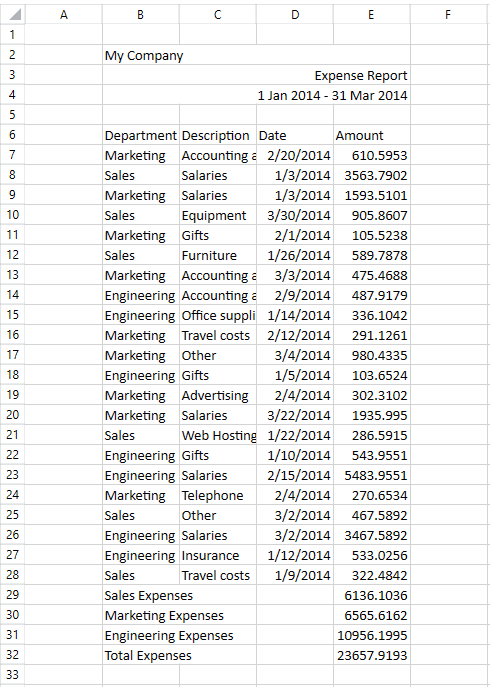To make the look of the workbook more consistent we can use the autofit feature on the columns containing data. In this way each of the columns will choose a width that fits its content and no data will be hidden or cropped.

In our example we can notice that some of the content is cropped due to insufficient column width. In order to see better what we have achieved for now we can fit the column widths so that none of the document content is cropped:

`worksheet.Columns[worksheet.UsedCellRange].AutoFitWidth();`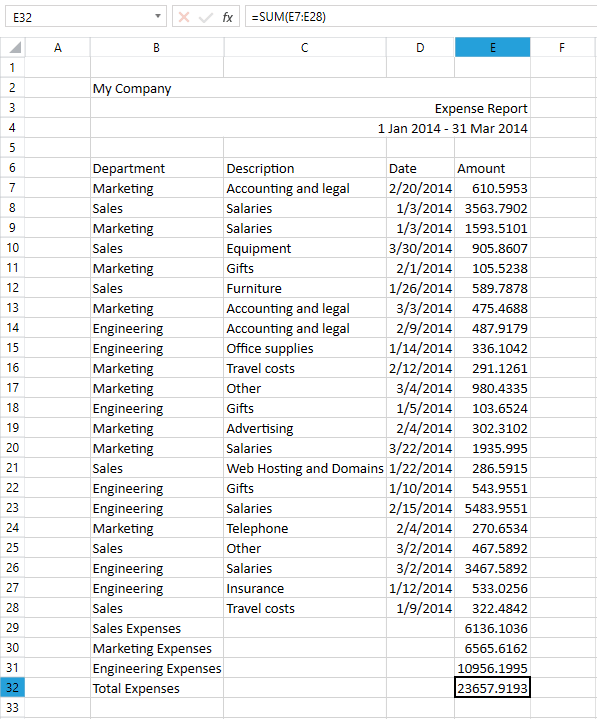That’s it! We have created a fully functional expense report from scratch. Stay tuned for the next two blog posts that show how this document can be easily styled, filtered and exported to PDF.

Now let's continue to part 2.